# How to Compare Two Columns in ExcelClaudia Buckley#### Join the Excel conversation on Slack

Ask a question or join the conversation for all things Excel on our Slack channel.

There are lots of reasons you might want to compare two columns in Excel for matches and differences. It might be that you want to eliminate or display duplicates to determine the frequency of an occurrence, or to highlight differences.

## How to compare two columns in Excel

Quick and easy: Match two columns and output a third

1. In a cell next to the two columns being compared, type an equal sign.
2. Enter the references of two cells to be compared, separated by an equal sign.
3. Hit Enter and copy that formula to the remaining rows.

Each value is compared with the value in the corresponding column. If they are the same, Excel returns a TRUE result. If they are not the same, the return value is FALSE.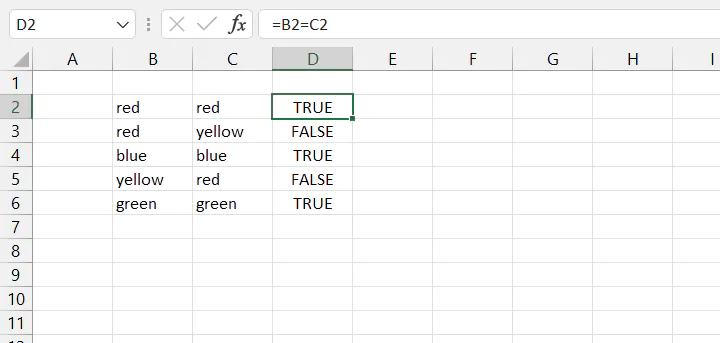## Examples

There are several options for comparing two lists, mainly because there are many different types of comparisons and desired outcomes. We’ve got lots of examples below.

### Example 1 - Helper column with TRUE/FALSE result

This example demonstrates the “quick and easy” method described above. We’ll compare Columns A and B using Column D as a helper column.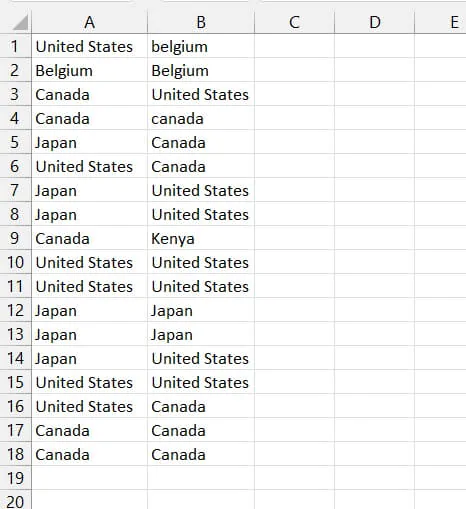1. In an empty column, enter the following formula in the first row:  =A1=B1
2. Copy that formula to the remaining rows. Because of relative cell referencing, the formula will adjust itself for each row.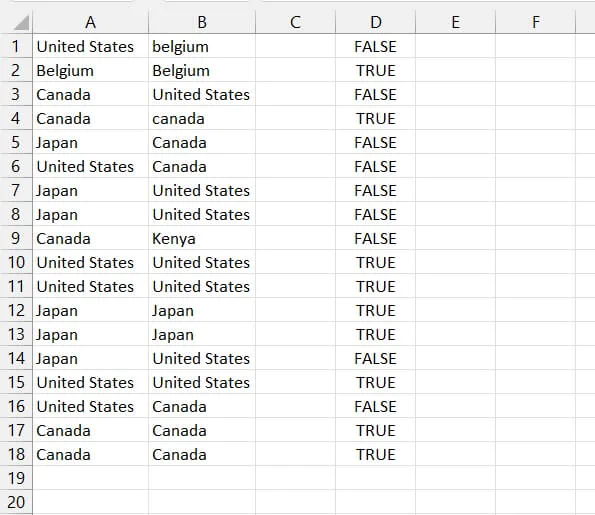If you have Excel 365 or later, this is a one-step solution because the result “spills” to all the cells in the range. Enter the following formula in the first row of the helper column:

``=A1:A18=B1:B18``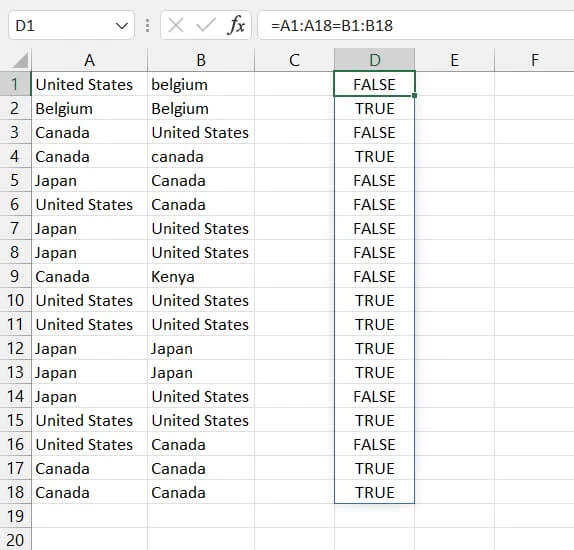Whether you’re using pre-dynamic or dynamic Excel, the result is the same. The formula in Column D compares each value in Column A with its Column B counterpart in the same row. A TRUE result means that the values are identical, and a FALSE result means they are not.

Note that this comparison is not case-sensitive, so “Canada” is considered identical to “canada” (see Row 4).

### Example 2 - compare two columns in Excel (case sensitive)

To compare two columns for case-sensitive duplicates, use the EXACT function.

Step 1 – Type the formula =EXACT(A1,B1) in a helper column

Step 2 – copy the formula to the remaining rows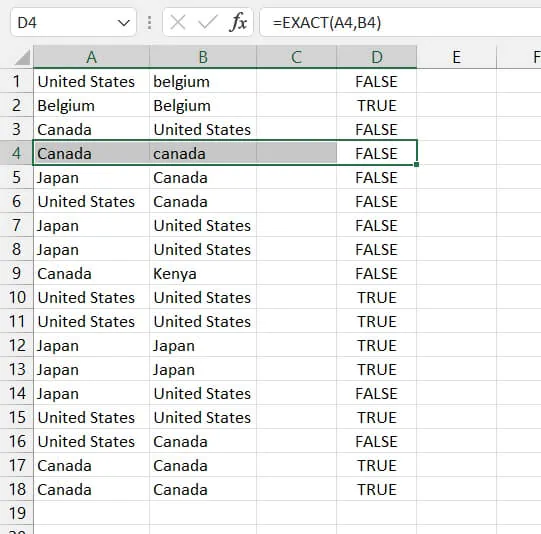### Example 3 - IF function (helper column with a customized response)

If you don’t like the TRUE/FALSE result given in the above examples, you can customize that response by using the IF function.

We can ask Excel to return the word “Match” when we compare row by row and find identical values.

``=IF(A1=B1, “Match”, “”)``

This means if the value in cell A1 is equal to the value in cell B1, return the text Match. Otherwise, display a blank cell. Note that text values are entered within double quotes, and that a blank cell is represented by two double quotes.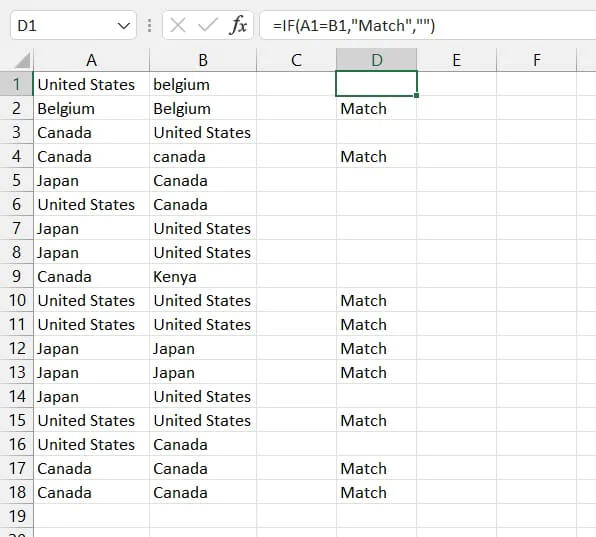### Example 4 - Conditional formatting (compare and highlight matches)

You can visually identify matching values in two rows by doing the following:

1. Highlight the two columns to be compared.
2. Go to the Home tab > Conditional Formatting on the ribbon.
3. Click New Rule.
4. In the New Formatting Rule Dialog box, choose “Use a formula to determine which cells to format.”
5. In the rule description field, enter =\$A1=\$B1. (Why the dollar signs?)
6. Click “Format…” to choose how to format cells that meet the condition.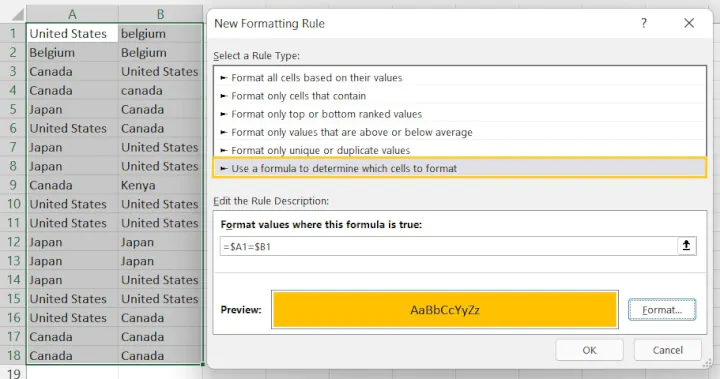7. Click OK.

Rows with matching values will be highlighted in the selected format.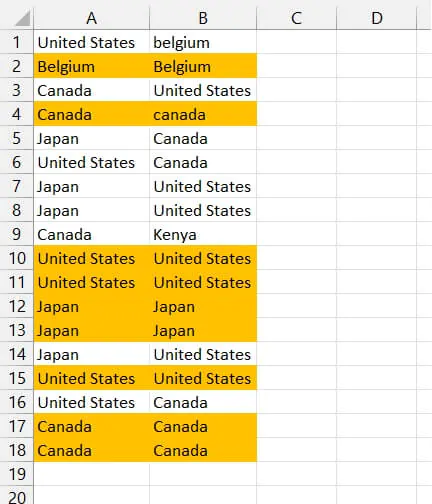To compare two columns using case-sensitive conditional formatting, use the EXACT function as described in Example 2.

### Example 5 - Conditional formatting (compare two lists and return differences)

To use conditional formatting to highlight differences in columns row by row, use the <> (is not equal to) operator instead of = (is equal to).

Rows where values do not match will be highlighted in the selected format. This method is not case sensitive, therefore “Canada” and “canada” are considered matching values and will not be highlighted.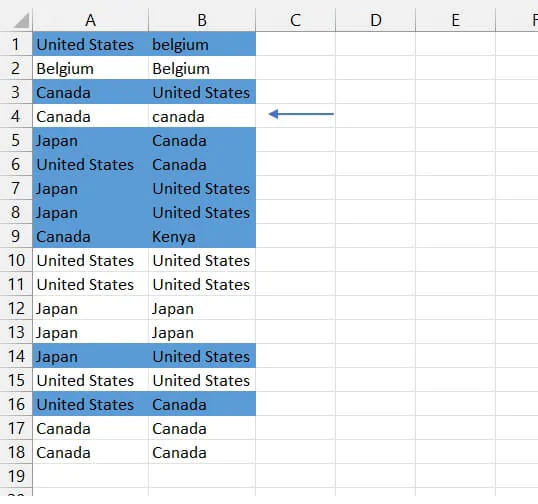### Example 6 - COUNTIF function (value appears anywhere in the other column)

If there’s a chance that the values to be compared may not line up row by row, use the COUNTIF function to compare columns in two lists in Excel where duplicates may be found anywhere in the list. Use the following steps.

1. In a helper column, enter the following formula:
``=COUNTIF(\$B\$1:\$B\$18,A1)``

This formula asks Excel to count the number of times the value found in cell A1 (learn more about relative cell references) is found in the absolute range B1:B18.

1. Copy the formula to the remaining rows
2. In an adjacent helper column, enter the following formula:
``=COUNTIF(\$A\$1:\$A\$18,B1)``

This formula asks Excel to count the number of times the value found in cell B1 is found in the absolute range A1:A18.

1. Copy the formula to the remaining rows.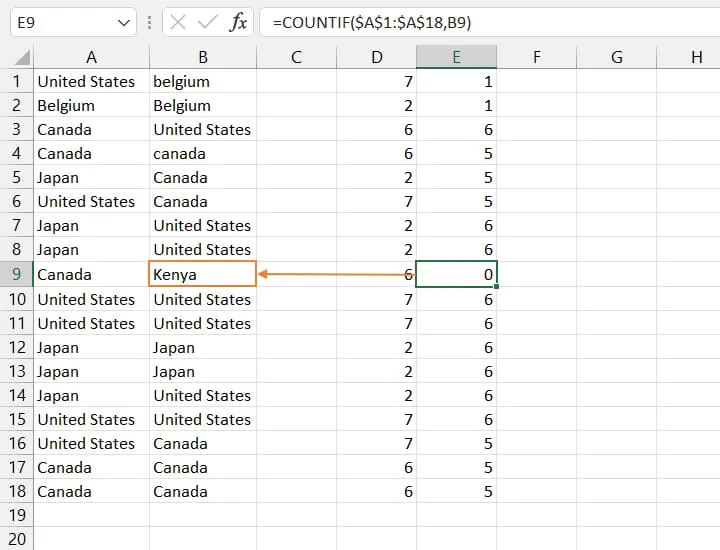In the example above, the value in cell B9 does not appear anywhere within the Column A range, so a count of zero is returned.

## Other ideas

As you can see, Excel can excel compare two lists and return matches or differences using several simple methods. With a little imagination, some of these ideas can be combined to create other solutions.

For example, use the UNIQUE function to generate two separate lists with unique or distinct values from each column, then compare for differences using the Duplicate Values option within the Conditional Formatting command.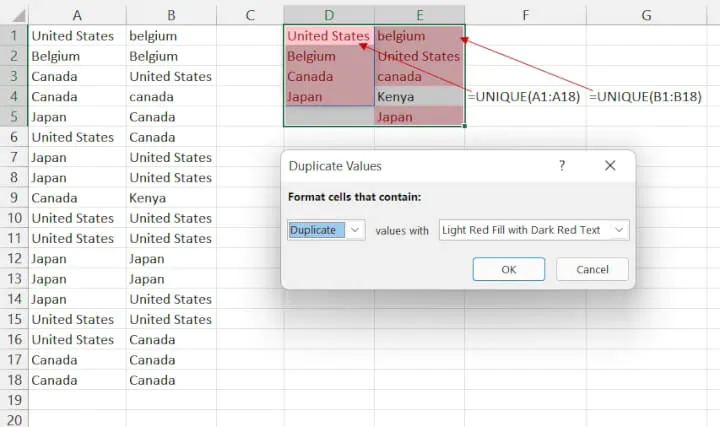Learn how to compare rows for duplicate values here.

Use Excel to get data-driven insights in our Excel for Marketers course. Try it for free!

Ready to become a certified Excel ninja?